# 3.9e: Exercises - Rational Functions

•• OpenStax
• OpenStax
$$\newcommand{\vecs}{\overset { \rightharpoonup} {\mathbf{#1}} }$$ $$\newcommand{\vecd}{\overset{-\!-\!\rightharpoonup}{\vphantom{a}\smash {#1}}}$$$$\newcommand{\id}{\mathrm{id}}$$ $$\newcommand{\Span}{\mathrm{span}}$$ $$\newcommand{\kernel}{\mathrm{null}\,}$$ $$\newcommand{\range}{\mathrm{range}\,}$$ $$\newcommand{\RealPart}{\mathrm{Re}}$$ $$\newcommand{\ImaginaryPart}{\mathrm{Im}}$$ $$\newcommand{\Argument}{\mathrm{Arg}}$$ $$\newcommand{\norm}{\| #1 \|}$$ $$\newcommand{\inner}{\langle #1, #2 \rangle}$$ $$\newcommand{\Span}{\mathrm{span}}$$ $$\newcommand{\id}{\mathrm{id}}$$ $$\newcommand{\Span}{\mathrm{span}}$$ $$\newcommand{\kernel}{\mathrm{null}\,}$$ $$\newcommand{\range}{\mathrm{range}\,}$$ $$\newcommand{\RealPart}{\mathrm{Re}}$$ $$\newcommand{\ImaginaryPart}{\mathrm{Im}}$$ $$\newcommand{\Argument}{\mathrm{Arg}}$$ $$\newcommand{\norm}{\| #1 \|}$$ $$\newcommand{\inner}{\langle #1, #2 \rangle}$$ $$\newcommand{\Span}{\mathrm{span}}$$$$\newcommand{\AA}{\unicode[.8,0]{x212B}}$$

### A: Conceptual Questions

Exercise $$\PageIndex{A}$$

1. What is the fundamental difference in the algebraic representation of a polynomial function and a rational function?

2. What is the fundamental difference in the graphs of polynomial functions and rational functions?

3. If the graph of a rational function has a removable discontinuity, what must be true of the functional rule?

4. Can a graph of a rational function have no vertical asymptote? If so, how?

5. Can a graph of a rational function have no x-intercepts? If so, how?

1. The rational function will be represented by a quotient of polynomial functions.
3. The numerator and denominator must have a common factor.
5. Yes. The numerator of the formula of the functions would have only complex roots and/or factors common to both the numerator and denominator.

### B: Find Domain of Rational Functions

Exercise $$\PageIndex{B}$$

$$\bigstar$$ Find the domain of the rational functions.

 7. $$f(x)=\dfrac{x+1}{x^{2}-1}$$ 8. $$f(x)=\dfrac{x-1}{x+2}$$ 9. $$f(x)=\dfrac{x^{2}+4 x-3}{x^{4}-5 x^{2}+4}$$ 10. $$f(x)=\dfrac{x^{2}+4}{x^{2}-2 x-8}$$

7. All reals except that $$x \neq -1,1$$
9. All reals except that $$x \neq-1,-2,1,2$$

### C: Find domain, vertical asymptotes, holes, and horizontal asymptotes

Exercise $$\PageIndex{C}$$

$$\bigstar$$ For each function, find (a) the domain, vertical asymptotes and holes, and (b) the horizontal asymptote and points of intersection of the HA with the function.

 $$f(x)=\dfrac{2x^2}{3x^2+1}$$ $$f(x)=\dfrac{4}{x-1}$$ $$f(x)=\dfrac{2x-2}{5x^2-3x-2}$$ $$f(x)=\dfrac{x+2}{x^{2}-9}$$ $$f(x)=\dfrac{x}{x^{2}+5 x-36}$$ $$f(x)=\dfrac{3+x}{x^{3}-27}$$ $$f(x)=\dfrac{3x-4}{x^{3}-16x}$$ $$f(x)=\dfrac{x^3-x}{x^3+9 x^2+14 x}$$ $$f(x)=\dfrac{x+5}{x^{2}-25}$$ $$f(x)=\dfrac{2x-4}{x-6}$$ $$f(x)=\dfrac{4-2x}{3 x-1}$$

$$\bigstar$$ Identify the removable discontinuity ("hole").

 $$f(x)=\dfrac{x^{2}-4}{x-2}$$ $$f(x)=\dfrac{x^{3}+1}{x+1}$$ $$f(x)=\dfrac{x^{2}+x-6}{x-2}$$ $$f(x)=\dfrac{2 x^{2}+5 x-3}{x+3}$$ $$f(x)=\dfrac{x^{3}+x^{2}}{x+1}$$

11. Domain is all reals;  no VA, No holes;      H.A. at $$y = \frac{2}{3}$$, No intersection;
13. Domain is all reals $$x \neq 1, -\frac{2}{5}$$, V.A. at $$x=-\frac{2}{5}$$; Hole at $$(1, \frac{2}{7} )$$;      H.A. at $$y=0$$; No intersection;
15. Domain is all reals $$x \neq 4,-9$$, V.A. at $$x=4,-9$$, No holes;      H.A. at $$y=0$$; Intersection at $$(0,0)$$;
17. Domain is all reals $$x \neq 0,4,-4$$, V.A. at $$x=0,4,-4$$, No holes;      H.A. at $$y=0$$; Intersection at $$(\frac{4}{3},0)$$;
19. Domain is all reals $$x \neq 5,-5$$, V.A. at $$x=5$$, Hole at $$(-5, \frac{-1}{10});$$      H.A. at $$y=0$$; No intersection;
21. Domain is all reals $$x \neq \frac{1}{3}$$, V.A. at $$x=\frac{1}{3}$$, No holes;      H.A. at $$y=-\frac{2}{3}$$; No intersection;
25. $$(2,4)$$     27. $$(2,5)$$     29. $$(-1,1)$$

### D: Describe local and end behaviour

Exercise $$\PageIndex{D}$$

$$\bigstar$$ Describe the local and end behavior of the functions.

 $$f(x)=\dfrac{x}{2 x+1}$$ $$f(x)=\dfrac{2x}{x-6}$$ $$f(x)=\dfrac{-2x}{x-6}$$ $$f(x)=\dfrac{x^{2}-4 x+3}{x^{2}-4x-5}$$ $$f(x)=\dfrac{2 x^{2}-32}{6 x^{2}+13x-5}$$

31. Local behavior: $$x \rightarrow-\frac{1}{2}^{+}, f(x) \rightarrow-\infty, x \rightarrow-\frac{1}{2}^{-}, f(x) \rightarrow \infty$$
End behavior: $$x \rightarrow \pm \infty, f(x) \rightarrow \frac{1}{2}$$

33. Local behavior: $$x \rightarrow 6^{+}, f(x) \rightarrow-\infty, x \rightarrow 6^{-}, f(x) \rightarrow \infty$$
End behavior: $$x \rightarrow \pm \infty, f(x) \rightarrow-2$$

35. Local behavior: $$x \rightarrow -\frac{5}{2}^{-}, \; f(x) \rightarrow-\infty, \quad x \rightarrow -\frac{5}{2}^{+}, \; f(x) \rightarrow \infty$$
$$x \rightarrow \frac{1}{3}^{-}, \; f(x) \rightarrow \infty, \quad x \rightarrow \frac{1}{3}^{+}, \; f(x) \rightarrow-\infty,$$ $$\quad$$       End behavior: $$x \rightarrow \pm \infty, f(x) \rightarrow \frac{1}{3}$$

### E: Find Slant Asymptote and its intersection with the function

Exercise $$\PageIndex{E}$$

$$\bigstar$$ For each function, (a) Find the slant asymptote of the functions, (b) Determine any points of intersection between the function and this asymptote.

 $$f(x)=\dfrac{24 x^{2}+6 x}{2 x+1}$$ $$f(x)=\dfrac{4 x^{2}-10}{2 x-4}$$ $$f(x)=\dfrac{81 x^{2}-18}{3 x-2}$$ $$f(x)=\dfrac{6 x^{3}-5 x}{3 x^{2}+4}$$ 40.   $$f(x)=\dfrac{x^{2}+5x+4}{x-1} \\$$ 40.1 $$f(x)=\dfrac{x^3-3x^2-x+3}{x^2-2x-8}$$

37. $$y=2 x+4$$ No point of intersection    39. $$y=2 x$$  Intersection at $$(0, 0)$$

### F: Find Intercepts

Exercise $$\PageIndex{F}$$

$$\bigstar$$ Find the $$x$$- and $$y$$-intercepts for the functions.

 43. $$f(x)=\dfrac{x}{x^{2}-x}$$ 44. $$f(x)=\dfrac{x+5}{x^{2}+4}$$ 45.  $$f(x)=\dfrac{x^{2}+x+6}{x^{2}-10 x+24}$$ 46.  $$f(x)=\dfrac{x^{2}+8 x+7}{x^{2}+11 x+30}$$ 48.  $$f(x)=\dfrac{94-2 x^{2}}{3 x^{2}-12}$$

43. none     45. $$x$$ -intercepts none, $$y$$ -intercept $$\left(0, \frac{1}{4}\right)$$

### G: Identify attributes and sketch

Exercise $$\PageIndex{G}$$

$$\bigstar$$ Find the (a) vertical asymptotes, (b) coordinates of any holes, (c) end behaviour asymptote (horizontal or slant), (d) coordinates of any points of intersection of the function with the end behaviour asymptote, (e) coordinates of any x-intercepts, (f) coordinates of the y-intercept. Use that information to sketch a graph.

 $$f(x)=\dfrac{4}{2x-3}$$ $$f(x)=\dfrac{2}{x+1}$$ $$p(x)=\dfrac{2x-3}{x+4}$$ $$q(x)=\dfrac{x-5}{3 x-1}$$ $$s(x)=\dfrac{4}{(x-2)^{2}}$$ $$r(x)=\dfrac{5}{(x+1)^{2}}$$ $$f(x)=\dfrac{x+2}{(x-1)(x-4)}$$ $$f(x)=\dfrac{2}{(x-1)(x+2)}$$ $$f(x)=\dfrac{(x-4)(x+3)^2}{(x+1)(x-4)(x-1)^2}$$ $$f(x) = \dfrac{ (x-2)(x+4)}{ (x-2)(x-3)(x+1)^2}$$ $$f(x) = \dfrac{(x-3)(x+6) }{ (x+2)(x+4)(x-1)(x+6)}$$ $$f(x) = \dfrac{ (x+1)(x-3)(-1)(x+2)}{ (x+1)(x-4)(x+3) }$$
 $$f(x)=\dfrac{3 x^{2}-14 x-5}{3 x^{2}+8 x-16}$$ $$g(x)=\dfrac{2 x^{2}+7 x-15}{3 x^{2}-14+15}$$ $$a(x)=\dfrac{x^{2}+2 x-3}{x^{2}-1}$$ $$b(x)=\dfrac{x^{2}-x-6}{x^{2}-4}$$ $$h(x)=\dfrac{2 x^{2}+x-1}{x-4}$$ $$k(x)=\dfrac{2 x^{2}-3 x-20}{x-5}$$ $$w(x)=\dfrac{(x-1)(x+3)(x-5)}{(x+2)^{2}(x-4)}$$ $$z(x)=\dfrac{(x+2)^{2}(x-5)}{(x-3)(x+1)(x+4)} \\[6pt]$$
 $$f(x) = \dfrac{ x^2+2}{ x}$$ $$f(x) = \dfrac{x^2+x+1 }{x+1 }$$ $$f(x) = \dfrac{ x^2-3x+2}{x-3 }$$ $$f(x) = \dfrac{ x^3+4x^2-x-4}{ x^2+2x-8}$$ $$f(x) = \dfrac{ x^2+3x+2}{ x-1}$$ $$f(x) = \dfrac{ x^2+3x+2}{ x+3}$$ $$f(x) = \dfrac{x^2+4x+3 }{ x+4}$$ $$f(x) = \dfrac{x^2-3x }{ x-4} \\[6pt]$$ $$f(x) = \dfrac{ x^2-4x+3}{x-2 }$$ $$f(x) = \dfrac{x^2-x-6 }{x-1 }$$ $$f(x) = \dfrac{x^2+2x-8 }{x+1 }$$ $$f(x) = \dfrac{ x^3-x^2-20x}{x^2+3x }$$ $$f(x) = \dfrac{4 x-12}{x^3-3x^2-4x+12 }$$ $$f(x) = \dfrac{2 }{ x^2-7x+10}$$ $$f(x) = \dfrac{x^2+9 }{x^2-16}$$ $$f(x) = \dfrac{ x^2-4}{x^2+2x-15 }$$
 $$f(x) = \dfrac{x^2-x }{ x^2+7x+10}$$ $$f(x) = \dfrac{x^2+4x+3 }{ x^2-5x+4}$$ $$f(x) = \dfrac{x^3-x^2-x+1 }{ 2x^2-11x+12}$$ $$f(x) = \dfrac{x^3-2x^2-x+2 }{ 3x^2-11x+6} \\[6pt]$$ $$f(x) = \dfrac{x-1 }{x^2-7x+10 }$$ $$f(x) = \dfrac{ x+2}{x^2+x-12 }$$ $$f(x) = \dfrac{ x^2-1}{x^3-x^2-4x+4 }$$ $$f(x) = \dfrac{ x^2+7x+12}{ x^3+9x^2+20x}$$ $$f(x) = \dfrac{x^2+4x+3 }{x^2+6x+8 }$$ $$f(x) = \dfrac{ x^2-2x-3}{x^2+2x }$$ $$f(x) = \dfrac{ x-3}{ x^2+x-2}$$ $$f(x) = \dfrac{x^2-6x+9 }{x^2+x-6 }$$ $$f(x) = \dfrac{ x^2+x-12}{ x^2-4}$$ $$f(x) = \dfrac{ x-3}{x^3-2x^2-4x+8 }$$ $$f(x) = \dfrac{ (x^3+3x^2+9x)}{ x^2+x-2}$$ $$f(x) = \dfrac{x^4+x^2 }{x^3-4x }$$
Answers to odd exercises #51-67. More graphs at the end of this section
 51. $$\left(\frac{3}{2}, \infty\right)$$53. V.A. $$x=-4$$, H.A. $$y=2$$; $$\left(\frac{3}{2}, 0\right)$$; $$\left(0,-\frac{3}{4}\right)$$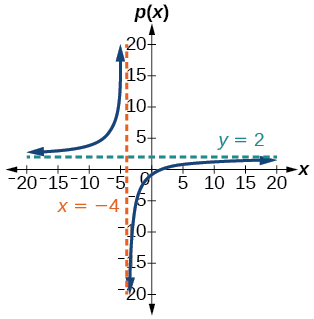55. V.A. $$x=2$$, H.A. $$y=0$$, $$(0,1)$$57. $$(-2,1) \cup(4, \infty)$$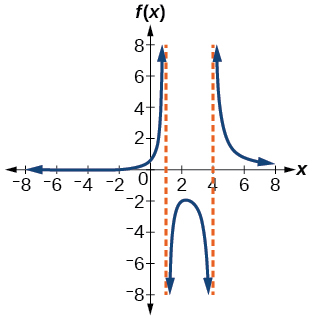59.61.63. V.A. $$x=-4$$, $$x=\frac{4}{3}$$, H.A. $$y=1$$; $$(5,0)$$; $$\left(-\frac{1}{3}, 0\right)$$; $$\left(0, \frac{5}{16}\right)$$65. V.A. $$x=−1$$, H.A. $$y=1$$; $$(−3,0)$$; $$(0,3)$$67. V.A. $$x=4$$, S.A. $$y=2x+9$$; $$(-1,0)$$; $$\left(\frac{1}{2}, 0\right)$$; $$\left(0, \frac{1}{4}\right)$$### H: Construct an Equation from a Description

Exercise $$\PageIndex{H}$$

$$\bigstar$$ Write an equation for a rational function with the given characteristics.

1. Vertical asymptotes at $$x=5$$ and $$x=-5$$, $$x$$-intercepts at $$(2,0)$$ and $$(-1,0)$$ , $$y$$ -intercept at $$(0,4)$$
2. Vertical asymptotes at $$x=-4$$ and $$x=-1, x$$ -intercepts at $$(1,0)$$ and $$(5,0), y$$ -intercept at $$(0,7)$$
3. Vertical asymptotes at $$x=-4$$ and $$x=-5, x$$ -intercepts at $$(4,0)$$ and $$(-6,0),$$ Horizontal asymptote at $$y=7$$
4. Vertical asymptotes at $$x=-3$$ and $$x=6, x$$ -intercepts at $$(-2,0)$$ and $$(1,0),$$ Horizontal asymptote at $$y=-2$$
5. Vertical asymptote at $$x=-1,$$ Double zero at $$x=2, y$$ -intercept at $$(0,2)$$
6. Vertical asymptote at $$x=3,$$ Double zero at $$x=1, y$$ -intercept at $$(0,4)$$

105. $$y=\dfrac{50(x-2)(x+1)}{(x-5)(x+5)}$$ $$\quad$$ 107. $$y=\dfrac{7(x+6)(x-4)}{(x+5)(x+4)}$$ $$\quad$$ 109. $$y=\dfrac{(x-2)^2}{2(x+1)}$$

### I: Construct an Equation from a Graph

Exercise $$\PageIndex{I}$$

$$\bigstar$$ Use the graphs to write an equation for the function.

 111.112.113.114.115.116.117.118.111. $$y= \dfrac{4(x-3)}{(x-4)(x+3)}$$ $$\quad$$ 113. $$y= \dfrac{27(x-2)}{(x+3)(x-3)^2}$$ $$\quad$$

115. $$y=\dfrac{(x+3)(x-2)}{3(x-1)}$$ $$\quad$$ 117. $$y=\dfrac{-6(x-1)^{2}}{(x+3)(x-2)^{2}}$$

### J: Use tables to show behaviour

Exercise $$\PageIndex{J}$$

$$\bigstar$$ Make tables to show the behavior of the function near the vertical asymptote and horizontal asymptote

 $$f(x)=\dfrac{1}{x-2}$$ $$f(x)=\dfrac{x}{x-3}$$ $$f(x)=\dfrac{2x}{x+4}$$ $$f(x)=\dfrac{2 x}{(x-3)^{2}}$$ $$f(x)=\dfrac{x^{2}}{x^{2}+2 x+1}$$
121.
Vertical asymptote $$x=2$$
2.01 2.001 2.0001 1.99 1.999
100 1,000 10,000 –100 –1,000
Horizontal asymptote $$y=0$$
10 100 1,000 10,000 100,000
.125 .0102 .001 .0001 .00001

123.
Vertical asymptote $$x=-4$$
–4.1 –4.01 –4.001 –3.99 –3.999
82 802 8,002 –798 –7998
Horizontal asymptote $$y=2$$
10 100 1,000 10,000 100,000
1.4286 1.9331 1.992 1.9992 1.999992

125.
Vertical asymptote $$x=-1$$
–.9 –.99 –.999 –1.1 –1.01
81 9,801 998,001 121 10,201
Horizontal asymptote $$y=1$$
10 100 1,000 10,000 100,000
.82645 .9803 .998 .9998 .99998

Exercise $$\PageIndex{A}$$

Graphs of odd-numbered exercises #71-101 in section G

69. V.A. $$x=-2$$, $$x=4$$, H.A. $$y=1$$, $$(1,0)$$; $$(5,0)$$; $$(-3,0)$$; $$\left(0,-\frac{15}{16}\right)$$71.73.75.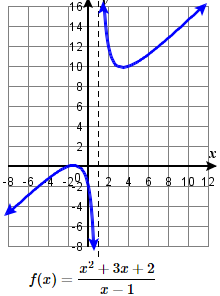77.79.81.83.85.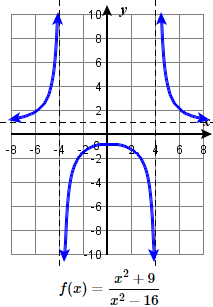87.89.91.93.95.97.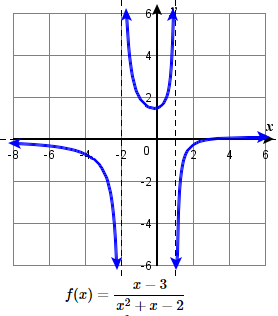99.101.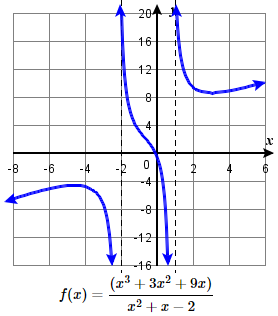$$\bigstar$$

This page titled 3.9e: Exercises - Rational Functions is shared under a CC BY 4.0 license and was authored, remixed, and/or curated by OpenStax.